##Artzt Parabolas of second kind

Consider a triangle ABC. Take the interior/exterior bisectors at angle C and the medial lines of the sides CA, CB. The four lines ares simultaneously tangent to a parabola qC. Analogously are defined two other parabolas qA, qB, corresponding to the other vertices of triangle ABC. These have, among others, the following properties:
 The focus of qC is on the symmedian from C at the corresponding vertex of the second Brocard triangle.
 The directrix of the parabola qC is the median from C.
 The line of middles B'C' of sides CA, CB is also tangent to qC.
 The medial line PN of the previous B'C' is also tangent to qC.
Parabolas like qC are called Artzt parabolas of second kind. There are also those of first kind examined in Artzt.html .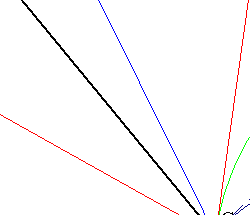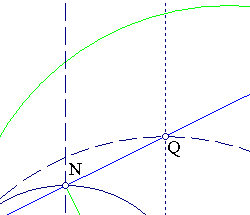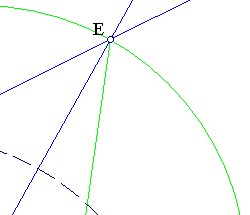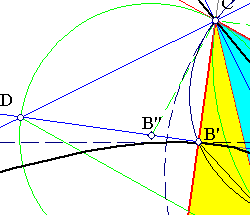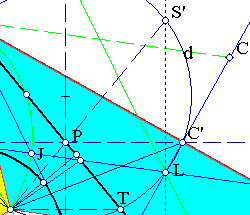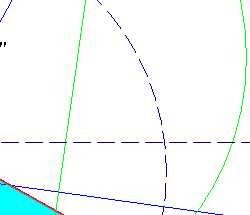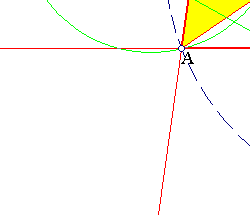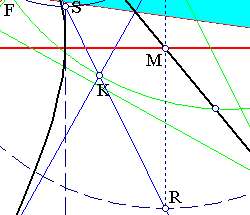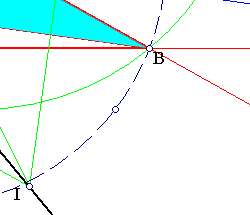According to Miquel (see Miquel_Point.html ) the focus F is the common intersection point of the circumcircles of the four triangles DCJ, CEK, DEL, JLK, from which the two first are right-angled at C, the third and fourth are isosceli.
- angle(B''CJ) = angle(B'JC) = pi/2 - C/2, implies that B''C is orthogonal to BC. Analogously CC'' is orthogonal to AC. Thus triangles ACF and CBF are similar with equal angles at F and angle(FBC) = angle(ACF). Besides angle(AFB) = 2*angle(C) and CF bisects angle(AFB). This characterizes the symmedian CF, and also F as the corresponding vertex of the second Brocard triangle.
C being on the directrix of the parabola qC implies that the symmetric of F with respect to the tangent CJ, which bisects angle C, is on the directrix too. Thus later coincides with the symmetric of the symmedian CF with respect to the bisector CJ, which identifies it with the median CM.
- Notice the equality of angle(ICB) = angle(ACF) = angle(FBC) hence the concurrence of lines C'C'', FB and CI at the same point. Similarly the concurrence of lines AF, B'B'', CI at the same point.
- Draw the diameter QR of the circumcircle which is orthogonal to side AB. The orthogonal to AB through P, middle of B'C', meets CE at point N, middle of DE (since P is the middle of CM), consequently quadrilateral CNC'L is cyclic, its circumcircle (d) being tangent to the circumcircle (has the radius LC as a diameter). Besides it passe through B', symmetric of C' with respect to NP. Also CK and NP intersect at S on (d).
- (d) passes through F. In fact FB', FC' being the medians of similar triangles AFC, CFB, implies that angle(B'FC') = angle(AFC) = pi-C.
- The symmetric of F with respect to PN is T, the intersection point of (d) with CP. In fact, CS being the bisector of angle(FCT) identifies T at this place. Since T is on the directrix PN is tangent to parabola qC.
- By the previous symmetry CFTS' is an isosceles trapezium whose angle of diagonals is bisected by lines NS and B'C'. Hence the symmetric of F with respect to B'C' is on the directrix CP. Thus B'C' is also tangent to the parabola qC.